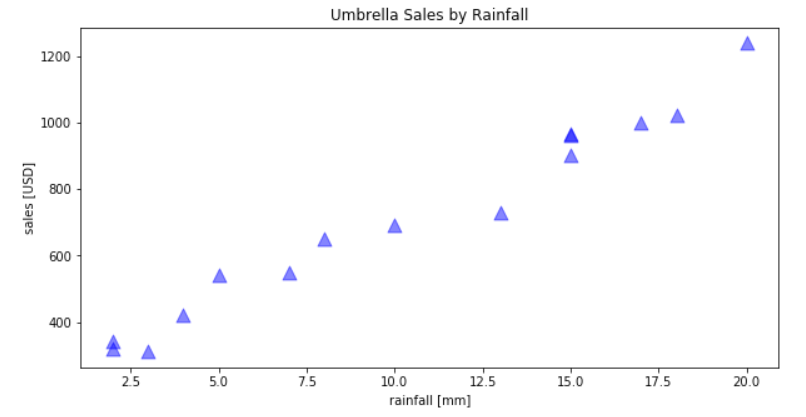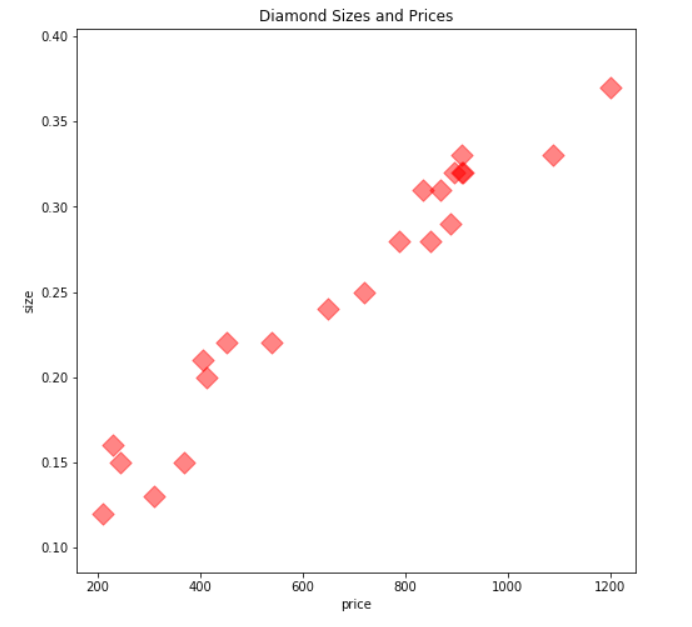Deals Of The Week - hours only!Up to 80% off on all courses and bundles.-Close
Introduction
Line plots
Multiple histograms
Other plot types
13. Scatter plots
Summary

## Instruction

Good job. Naturally, matplotlib offers numerous other types of plots. Scatter plots are a frequent choice for many analysts. Creating them with matplotlib is very similar to creating histograms or line plots, so we won't explain everything from scratch here. Instead, take a look at the example below:umbrellas = pd.read_csv('umbrellas.csv')
scatter_figure = plt.figure(figsize=(10, 5))
scatter_subplot = plt.subplot(111)
scatter_subplot.set_title('Umbrella Sales by Rainfall')
scatter_subplot.set_xlabel('rainfall [mm]')
scatter_subplot.set_ylabel('sales [USD]')

plt.scatter(umbrellas['rainfall'], umbrellas['sales'], c='blue', alpha=0.5, s=120, marker='^')


Take a look at the last line above. Our plt.scatter takes the following two obligatory arguments:

1. umbrellas['rainfall'] – x-axis data,
2. umbrellas['sales'] – y-axis data.

We also added some optional arguments:

1. c='blue' means our points will be of colored blue,
2. alpha=0.5 means our points will be 50% transparent,
3. s=120 defines the size of our points,
4. marker='^' means that each point will be shown as a triangle.

## Exercise

It's time for you to create your own scatter plot! Use diamond_prices.csv, which contains data about diamond sizes and their prices, and draw the following plot:Hints:

• Use figsize=5x5.
• For plot points, use a "red" color with 50% transparency, a size of "100" and a marker "D".
• Use the plt.subplot(111).

### Stuck? Here's a hint!

Once you import the csv file, and create the subplot and the axes, you can draw the scatter plot with:

plt.scatter(diamonds['price'], diamonds['size'], c='r', alpha=0.5, s=100, marker='D')